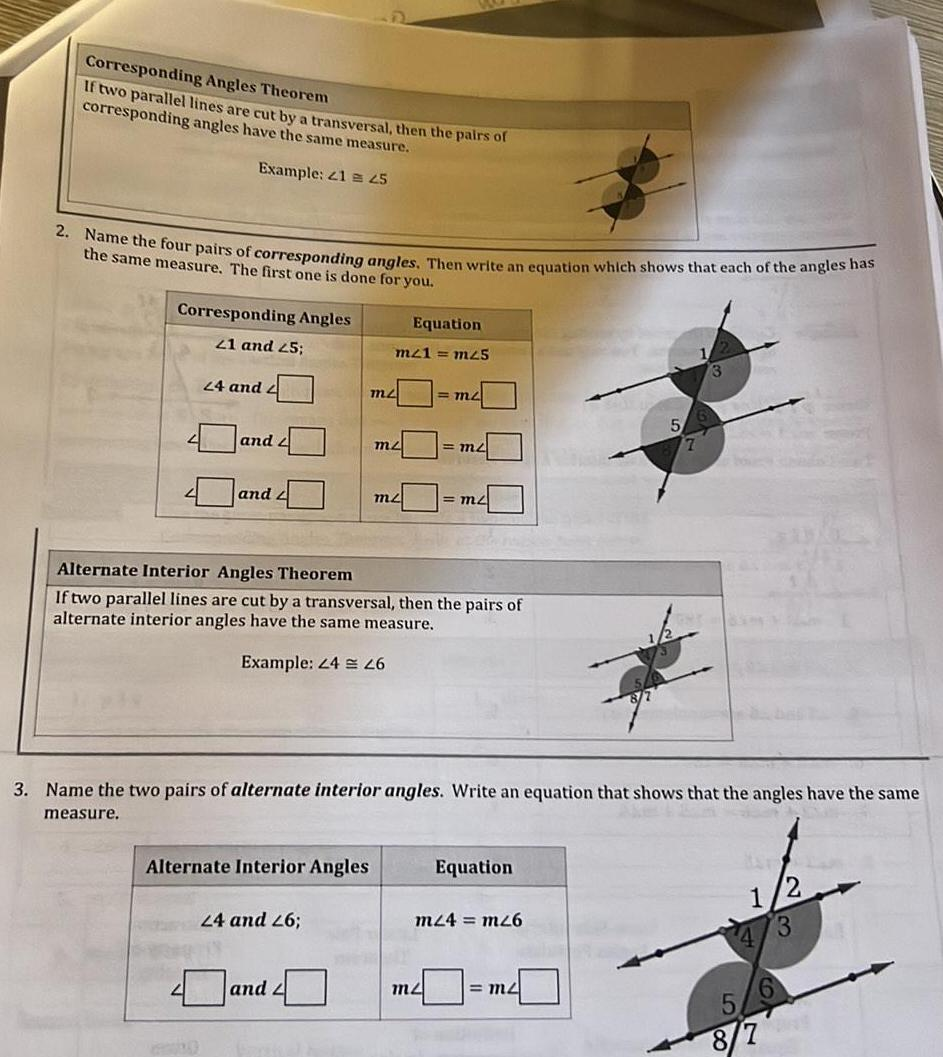Question:

# Corresponding Angles Theorem If two parallel lines are cut

Last updated: 11/20/2023Corresponding Angles Theorem If two parallel lines are cut by a transversal then the pairs of corresponding angles have the same measure Example 21 45 2 Name the four pairs of corresponding angles Then write an equation which shows that each of the angles has the same measure The first one is done for you Corresponding Angles 21 and 25 24 and 2 and 2 and Alternate Interior Angles Equation m21 m25 24 and 26 m and mz mz Alternate Interior Angles Theorem If two parallel lines are cut by a transversal then the pairs of alternate interior angles have the same measure Example 24 26 m2 1 m2 3 Name the two pairs of alternate interior angles Write an equation that shows that the angles have the same measure m2 Equation m24 mz6 5 m2 7 2 1 4 3 8 7